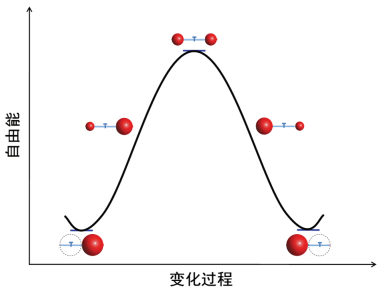## 通过物理化学研讨课培养学生的研究能力——以两个连通气泡的变化为例,1, 1, 1, 1, 1, 1, 1, 1, 2

## Cultivating Students' Research Ability in the Physical Chemistry Seminar,1, 1, 1, 1, 1, 1, 1, 1, 2

 基金资助: 物理化学在线开放课程群建设的创新与实践项目.  16ZG004-14

 Fund supported: 物理化学在线开放课程群建设的创新与实践项目.  16ZG004-14Abstract

In the physical chemistry course, surface tension, surface free energy, and Young-Laplace equation are the basics in interface and colloidal chemistry. For students, it is the key to understand the above mentioned content for studying the follow-up knowledge. This paper presented the physical chemistry seminar via exploring the changes of two connected bubbles with different sizes. The demonstration experiments were valuable for students to know better surface tension, surface free energy, and Young-Laplace equation. Cultivating students' research abilities to apply knowledge and analyze problems were also based on discussing the similarities and differences between two connected balloon and two connected bubbles. The problem-based seminar on physical chemistry could be significant for the related courses.

Keywords： Physical chemistry ; Seminar ; Bubble experiment ; Cultivating students' ability

YUAN Hong, WANG Ruifang, LI Lei, TONG Jiawen, YANG Jian, SHI Junyang, LI Gang, LI Wuke, HUANG Jin. Cultivating Students' Research Ability in the Physical Chemistry Seminar. University Chemistry[J], 2018, 33(5): 10-17 doi:10.3866/PKU.DXHX201712035

### 图1(a)左边气泡半径为r1，右边气泡半径为r2；(b)两侧气泡的半径为r3；连通两个气泡的细管的内半径为r0，旋塞到左边端口的长度为l1，旋塞到右边端口的长度为l2

### 图2### 图3#### 1.2 从整体的角度进行分析

$\frac{{{p_1}{V_1}}}{{{n_1}}} = RT$，其中${V_1} = \frac{4}{3}{\rm{ \mathit{ π} }}r_1^3 + {l_1} \times {\rm{ \mathit{ π} }}r_0^2$${p_1} = \frac{{4\sigma }}{{{r_1}}} + {p_0} 对右边的气体(气体的体积为V2，摩尔数为n2)有： \frac{{{p_2}{V_2}}}{{{n_2}}} = RT，其中{V_2} = \frac{4}{3}{\rm{ \mathit{ π} }}r_2^3 + {l_2} \times {\rm{ \mathit{ π} }}r_0^2$${p_2} = \frac{{4\sigma }}{{{r_2}}} + {p_0}$

$\frac{{{p_3}{V_3}}}{{{n_3}}} = RT$，其中${V_3} = \frac{4}{3}{\rm{ \mathit{ π} }}r_3^3 + ({l_1} + {l_2}) \times {\rm{ \mathit{ π} }}r_0^2$${p_3} = \frac{{4\sigma }}{{{r_3}}} + {p_0}$

### 图4## 2 教学过程的设计及实施

### 图5## 参考文献 原文顺序 文献年度倒序 文中引用次数倒序 被引期刊影响因子

[2018-02-04]. http://v.ifeng.com/video_3841860.shtml.

/

 〈〉